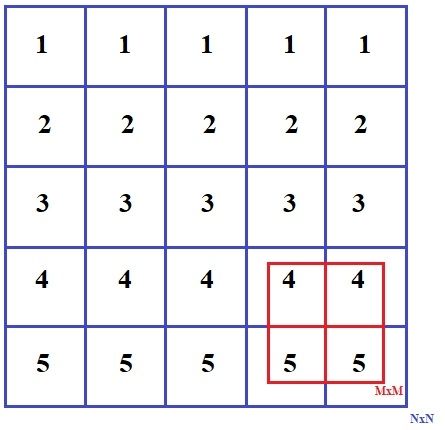# Print maximum sum square sub-matrix of given size in C Program.

Given a matrix of NxN find a sub matrix of MxM where M<=N and M>=1 such that addition of all the elements of matrix MxM is maximum. Input of matrix NxN can contain zero, positive and negative integer values.## Example

Input:
{{1, 1, 1, 1, 1},
{2, 2, 2, 2, 2},
{3, 3, 3, 3, 3},
{4, 4, 4, 4, 4},
{5, 5, 5, 5, 5} }
Output:
4 4
5 5

The above problem can be solved by a simple solution in which we can take whole matrix NxN, then find out all possible MxM matrix and find their sum, then print the one matrix of MxM with the maximum sum. This approach is easy but require O(N^2.M^2) time complexity, so we try to find out a way which takes less time complexity.

## Algorithm

Start
Step 1 -> Declare Function void matrix(int arr[][size], int k)
IF k>size
Return
Declare int array[size][size]
Loop For int j=0 and j<size and j++
Set sum=0
Loop for int i=0 and i<k and i++
Set sum=sum + arr[i][j]
End
Set array[j]=sum
Loop For int i=1 and i<size-k+1 and i++
Set sum=sum+(arr[i+k-1]][j]-arr[i-1][j]
Set arrayi][j]=sum
End
Set int maxsum = INT_MIN and *pos = NULL
Loop For int i=0 and i<size-k+1 and i++)
Set int sum = 0
Loop For int j = 0 and j<k and j++
Set sum += array[i][j]
End
If sum > maxsum
Set maxsum = sum
Set pos = &(arr[i])
End
Loop For int j=1 and j<size-k+1 and j++
Set sum += (array[i][j+k-1] - array[i][j-1])
IF sum > maxsum
Set maxsum = sum
Set pos = &(arr[i][j])
End
End
End
Loop For int i=0 and i<k and i++
Loop For int j=0 and j<k and j++
Print *(pos + i*size + j)
End
Print
End
Step 2 -> In main()
Declare int array[size][size] = {{1, 1, 1, 1, 1}, {2, 2, 2, 2, 2}, {3, 3, 3, 3, 3}, {4, 4, 4, 4, 4}, {5, 5, 5, 5, 5}}
Declare int k = 2
Call matrix(array, k)
Stop

## Example

#include <bits/stdc++.h>
using namespace std;
#define size 5
void matrix(int arr[][size], int k){
if (k > size) return;
int array[size][size];
for (int j=0; j<size; j++){
int sum = 0;
for (int i=0; i<k; i++)
sum += arr[i][j];
array[j] = sum;
for (int i=1; i<size-k+1; i++){
sum += (arr[i+k-1][j] - arr[i-1][j]);
array[i][j] = sum;
}
}
int maxsum = INT_MIN, *pos = NULL;
for (int i=0; i<size-k+1; i++){
int sum = 0;
for (int j = 0; j<k; j++)
sum += array[i][j];
if (sum > maxsum){
maxsum = sum;
pos = &(arr[i]);
}
for (int j=1; j<size-k+1; j++){
sum += (array[i][j+k-1] - array[i][j-1]);
if (sum > maxsum){
maxsum = sum;
pos = &(arr[i][j]);
}
}
}
for (int i=0; i<k; i++){
for (int j=0; j<k; j++)
cout << *(pos + i*size + j) << " ";
cout << endl;
}
}
int main(){
int array[size][size] = {
{1, 1, 1, 1, 1},
{2, 2, 2, 2, 2},
{3, 3, 3, 3, 3},
{4, 4, 4, 4, 4},
{5, 5, 5, 5, 5},
};
int k = 2;
matrix(array, k);
return 0;
}

## Output

if we run above program then it will generate following output

4 4
5 5